Question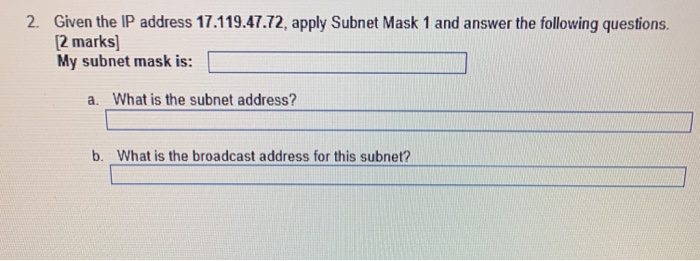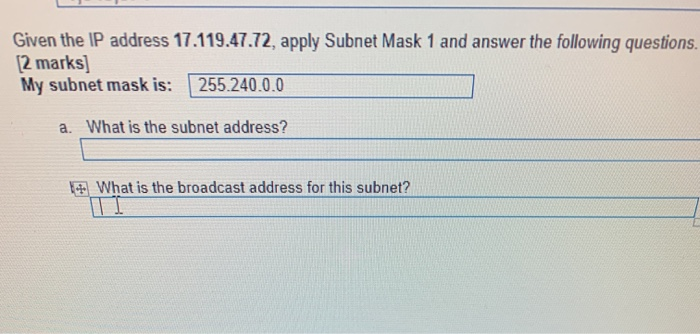Here IP is 17.119.47.72 (in binary  00010001 01110111 00101111 01001000)

Subnet mask is 255.240.0.0 ( in binary 11111111 11110000 00000000 00000000)

you need to perform a bit-wise AND operation (1+1=1, 1+0 or 0+1 =0, 0+0=0) on the host IP address and subnet mask

00010001 01110111 00101111 01001000 ( IP address)

AND 11111111 11110000 00000000 00000000 ( Subnet mask)

= 00010001 01110000 00000000 00000000 (Network address)

00010001 01110000 00000000 00000000 (Network address)

OR 00000000 00001111 11111111 11111111 ( Inverted Subnet mask )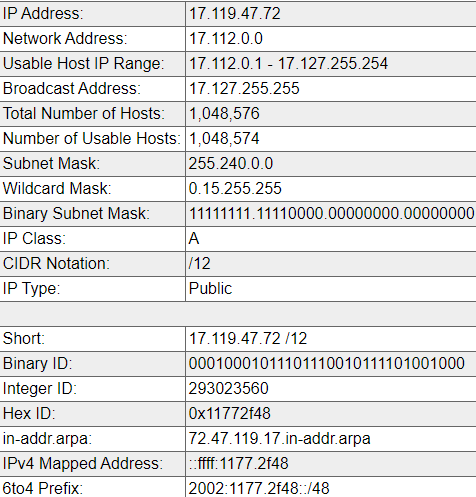Hope you like it !

Earn Coins

Coins can be redeemed for fabulous gifts.

Similar Homework Help Questions
• Given the following IP address and subnet mask, what is the network identifier? • IP Address:...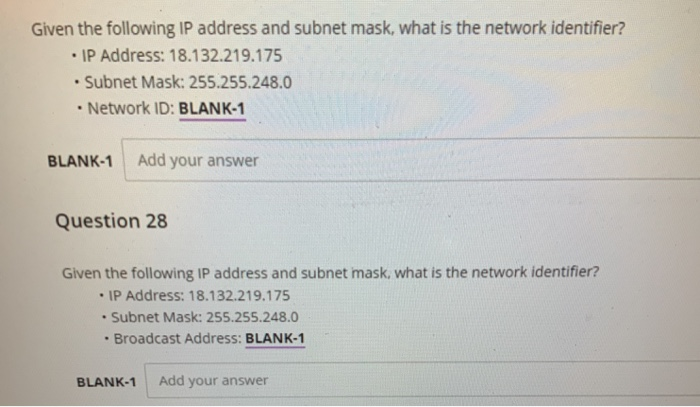Given the following IP address and subnet mask, what is the network identifier? • IP Address: 18.132.219.175 . Subnet Mask: 255.255.248.0 Network ID: BLANK-1 BLANK-1 Add your answer Question 28 Given the following IP address and subnet mask, what is the network identifier? - IP Address: 18.132.219.175 . Subnet Mask: 255.255.248.0 • Broadcast Address: BLANK-1 BLANK-1 Add your answer

• using the information given below . what is the ip address , subnet mask , default...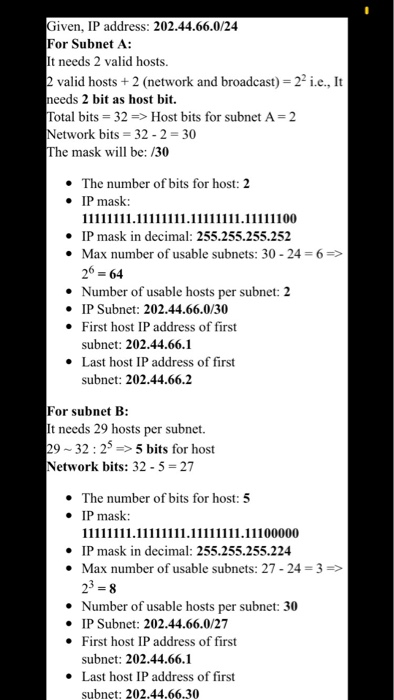using the information given below . what is the ip address , subnet mask , default gateway? ** corrections # of bits in subnet for A :6 # of bits in submet for B: 3 PC-A R1 G0/0 R GO/1 S1 PC-B ? assign the IP ADDRESS information with given information Given, IP address: 202.44.66.0/24 For Subnet A: It needs 2 valid hosts 2 valid hosts + 2 (network and broadcast) = 22 i.e., It needs 2 bit as host...

Given an IP address and mask of 192.168.10.0/24 255.255.255.0 (address / mask) subnet A has 100 hosts subnet b has 50 hosts Specification Subnet A Subnet B Number of bits in the subnet IP mask (binary) New IP mask (decimal) Maximum number of usable subnets (including the 0th subnet) Number of usable hosts per subnet IP Subnet First IP Host address Last IP Host address Description Subnet A Subnet B First IP address Last IP address Maximum number of hosts

• 4. Given a network IP address of 130.124.0.0 answer the following questions if 8 subnets are...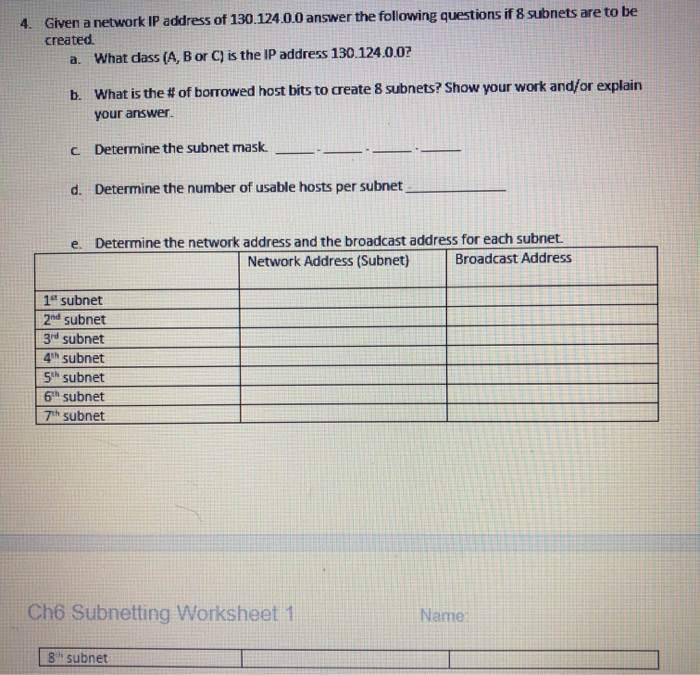4. Given a network IP address of 130.124.0.0 answer the following questions if 8 subnets are to be created a. What dass (A, B or C) is the IP address 130.124.0.07 b. What is the # of borrowed host bits to create 8 subnets? Show your work and/or explain your answer. c Determine the subnet mask d. Determine the number of usable hosts per subnet e. Determine the network address and the broadcast address for each subnet. Network Address (Subnet)...

• Network IP Addressing Network Layout of 2 networks Given an IP address and mask of 172.23.29.0/24,...

Network IP Addressing Network Layout of 2 networks Given an IP address and mask of 172.23.29.0/24, design an IP addressing scheme that satisfies the following requirements. Subnet B Specification This network requires space for 62 machines plus the router Number of bits in the subnet Blank 1 IP mask (binary) Blank 2 New IP mask (decimal) Blank 3 Maximum number of usable subnets of this size when using this mask (including the 0th subnet) Blank 4 Number of usable hosts...

• In a class B subnet, we know the IP address of one of the hosts and...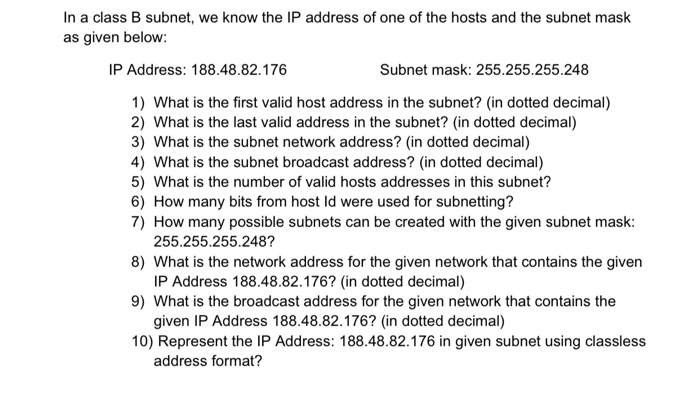In a class B subnet, we know the IP address of one of the hosts and the subnet mask as given below: IP Address: 188.48.82.176 Subnet mask: 255.255.255.248 1) What is the first valid host address in the subnet? (in dotted decimal) 2) What is the last valid address in the subnet? (in dotted decimal) 3) What is the subnet network address? (in dotted decimal) 4) What is the subnet broadcast address? (in dotted decimal) 5) What is the number...

• You are given a class B IP Address of 169.33.0.0 and you need to create a network with at least 20 subnets and at l...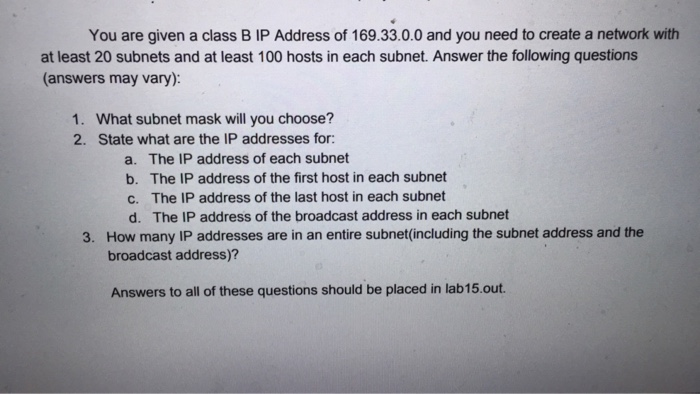You are given a class B IP Address of 169.33.0.0 and you need to create a network with at least 20 subnets and at least 100 hosts in each subnet. Answer the following questions (answers may vary): 1. What subnet mask will you choosse? 2. State what are the IP addresses for: a. The IP address of each subnet b. The IP address of the first host in each subnet c. The IP address of the last host in each...

• Given an IP address and mask of 192.168.1.0 255.255.255.0 (address / mask) subnet A has 55 hosts subnet b has 112 hosts The 0th subnet is not used. (Do not use 192.168.1.0) (Subnetting Help) Subne...

Given an IP address and mask of 192.168.1.0 255.255.255.0 (address / mask) subnet A has 55 hosts subnet b has 112 hosts The 0th subnet is not used. (Do not use 192.168.1.0) (Subnetting Help) Subnet A Specification Student Input Number of Network bits in the subnet mask New Subnet mask (decimal) Number of usable hosts IP Subnet (Network ID) First IP Host address Last IP Host address (Subnetting Help) Subnet B Specification Student Input Number of Network bits in the...

• Scenario: IP Address: 192.168.1.0 Subnet Mask: 255.255.255.? What is the subnet mask need to be if...

Scenario: IP Address: 192.168.1.0 Subnet Mask: 255.255.255.? What is the subnet mask need to be if we want to break up the IP Address space into 4 separate networks??

NETWORKING I please help.. thanks you. Given that a subnet mask byte value has bits set to 1 from left to right there is a small set of possible values. The first tow values are 128,192. What are the rest of the values? Hint: here are place values or each bit in a byte 128           64          32           16            8            4         2          1 What are the rest of the values? 128, 192... Consider the following pc configuration IP address:                 192.168.1.2 Subnet...